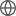Old Web
English
In Dou et al. (2021), we introduced the Fundamental Formation Relation (FFR), a tight relation between specific SFR (sSFR), H$_2$ star formation efficiency (SFE$_{\rm H_2}$), and the ratio of H$_2$ to stellar mass. Here we show that atomic gas HI does not follow a similar FFR as H$_2$. The relation between SFE$_{\rm HI}$ and sSFR shows significant scatter and strong systematic dependence on all of the key galaxy properties that we have explored. The dramatic difference between HI and H$_2$ indicates that different processes (e.g., quenching by different mechanisms) may have very different effects on the HI in different galaxies and hence produce different SFE$_{\rm HI}$-sSFR relations, while the SFE$_{\rm H_2}$-sSFR relation remains unaffected. The facts that SFE$_{\rm H_2}$-sSFR relation is independent of other key galaxy properties, and that sSFR is directly related to the cosmic time and acts as the cosmic clock, make it natural and very simple to study how different galaxy populations (with different properties and undergoing different processes) evolve on the same SFE$_{\rm H_2}$-sSFR $\sim t$ relation. In the gas regulator model (GRM), the evolution of a galaxy on the SFE$_{\rm H_2}$-sSFR($t$) relation is uniquely set by a single mass-loading parameter $\lambda_{\rm net,H_2}$. This simplicity allows us to accurately derive the H$_2$ supply and removal rates of the local galaxy populations with different stellar masses, from star-forming galaxies to the galaxies in the process of being quenched. This combination of FFR and GRM, together with the stellar metallicity requirement, provide a new powerful tool to study galaxy formation and evolution.